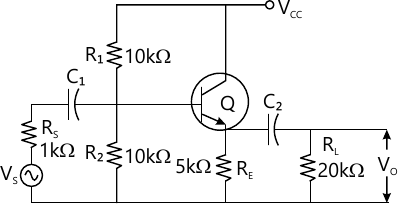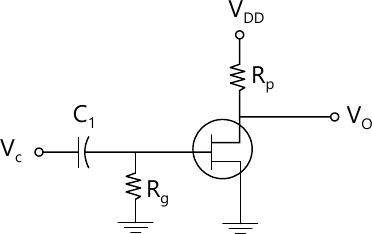MORE IN Analog Electronics
Total marks: --
Total time: --
INSTRUCTIONS
(1) Assume appropriate data and state your reasons
(2) Marks are given to the right of every question
(3) Draw neat diagrams wherever necessary

1(a) Explain the following:
i) practical diode model.
ii) ideal diode model.
iii) piecewise linear model.
8 M
1(b) Consider a half wave and full wave rectifier with capacitor input dieter.Derive an expression for ripple factor.
8 M
1(c) explain the oration of negative circuit.
4 M

2(a) consider a fixed bias circuit of a transistor.Obtain expressions for stability factor Sico,Svbe and $s_{b}$.Draw the circuit diagram.
10 M
2(b) Design n voltage divider bias circuit for the given conditions.$I_{c}=1mA,\ S_{ico}-20,\beta -100,V_{e}-1\V,V_{ce}-6V\ and \ V_{cc}-12V.$ Draw the circuit diagram.
10 M

3(a) For the common collector circuit shown in fig.q3 (a) the transistor h-parameters are $h_{fe}=-101,h_{re}=1,h_{oc-25\mu \ A/V,},h_{ie}=1.2k.$Determine $R_{1},A_{i}\ A_{v,}\A\ A_{vs}\ and\ R_{o}$circuit.10 M
3(b) State and prove miller's theorem.
5 M
3(c) Obtain r-parameter model for GB mode.
5 M

4(a) Explain the low frequency response of single stage RC coupled amplifier.
10 M
4(b) an amplifier consists of 3 identical stages in cascade,the bandwidth of overall amplifier extends form 20 Hz to 20 kHz.Determine the bandwidth of individual stage.
5 M
4(c) For an amplifier,the midland again is 100 and lower cut-off frequency is 1 kHz.calculate the again of the amplifier at frequency of 20 Hz.
5 M

5(a) consider Darling ton emitter follower circuit.Obtain expressions for $R_{i2},R_{i1},R_{i2},A_{i1}\\ compare \ R_{i}\ and \ A_{i}/] of each and overall stage if \[R_{e}=3.3k\Omega ,h_{re}-2.5X10^{-4},h_{fe}=50\\ and \ h_{oe}=25\mu \A\V.$
10 M
5(b) For a voltage series feedback amplifier topology,obtain expression for$A_{v} and R_{if}.$ Also explain the principle of voltage amplifier used in feedback amplifiers.
10 M

6(a) Derive an expression for second harmonic distortion in power amplifiers,using 3-point method.
10 M
6(b) a complementary symmetry push pull amplifier is operated with $V_{cc}$=10V,$R_{L}=5\Omega$. Determine maximum output power,power rating of transistors and DC input power.
10 M

7(a) Explain the concept of positive feedback used in oscillators.
5 M
7(b) obtain an expression for frequency of oscillation in Colpitt's oscillator.
10 M
7(c) in a RC phase shift oscillator using transistor,
$F_{0}=10 kHz,R_{1}=25k\Omega ,R_{2}=57, \[R_{1}=5\Omega$. Calculate the capacitor C and $h_{fe}$.Draw the circuit diagram.
5 M

8(a) Consider a n-channel JEET using voltage divider bias. Explain its DC analysis, Also derive an expression for transconductance gm.
10 M
8(b) Design a fixed bias circuit of fig.q8 (b) to have ac gain of -15.Calculate the value of $R_{d}\ to \get\ get\ this\ again,\ if V_{dd}=40V,R_{g}-10\ M\Omega ,I_{dss}-10mA,V_{p}\\ =-4v,Yos=20\mu s,C_{1}=0.1\mu F.$10 M

More question papers from Analog Electronics